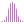#Average (PQL)

Returns the average for the elements in the specified list.

#### Syntax

Average( <List> , OPTIONAL )

###### Syntax Alternatives

Avg( <List> , OPTIONAL )

* Click on the function's arguments above for more details on the input values.

• List parameter should be a list or set of elements from an existing hierarchy in the model.
• The data point or tuple is optional, and describes the values used to generate the average. If not supplied, the default measure or the query context will determine this value instead.
• For details on how to employ and use this function see the semantic calculation overview.
##### Different Function types
• This function is like the MDX average function; it calculates an average across a list of elements in a hierarchy or attribute.
• If, instead, you wish to change the aggregate of a numeric column in the database to average, use this function
• To use a simple average mathematical operation on a list of numbers, use the Common library function.

#### Examples

This example returns the average of expenses by product category in the product table/ dimension, using the sample demo model:

Average( {AllMembers([products].[Product Category])}, ([measures].[data Expenses]) )

Below, using the sample data of the 4 product categories we can use the above formula to calculate the average. Totaling the 4 items and dividing by 4 produces an average if 2,549,357: